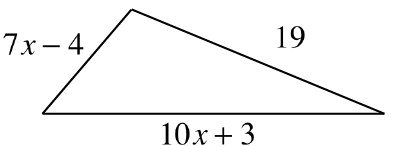### Home > GB8I > Chapter 3 Unit 4 > Lesson INT1: 3.1.4 > Problem3-47

3-47.

The perimeter of the triangle at right is $52$ units. Write and solve an equation based on the information in the diagram. Use your solution for $x$ to calculate the length of each side of the triangle. Be sure to confirm that your answer is correct.Find the sum of the side lengths.

The sum of the side lengths is the perimeter, which we know from the problem is $52$ units.Set expression from Step 1 equal to $52$ and solve for $x$.

$(7x − 4) + (19) + (10x + 3)$

$(7x − 4) + (19) + (10x + 3) = 52$
$17x + 18 = 52$

Continue solving finding the value of $x$.

Find side lengths substituting your found value of $x$ into the expression given for each side.